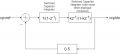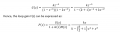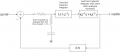# Combining s and z-domain Mathematics in Analogue Switched-Capacitor Circuit

Joined Oct 30, 2017
24
Hello!

At present I'm trying to implement a DC-DC converter control system-type circuit in CMOS. As part of this, I'm trying to work through some mathematics and create transfer functions of the circuit.

Part of the circuit is implemented in discrete-time, switched capacitor circuits; however, it is necessary for various reasons to have passive components forming poles and zeroes at various nodes at low enough frequency to effect the behavior of the discrete time components. How would I model this, as I would assume the SC circuit requires z-domain and the passive filters require s-domain??

#### LvW

Joined Jun 13, 2013
1,428
To me it is not quite clear what your problem is.The system you are going to develop - is it a mixture between passiv/analog and switched-capacitor? More than that, are you asking for models only - or for realization hints?

Joined Oct 30, 2017
24
To me it is not quite clear what your problem is.The system you are going to develop - is it a mixture between passiv/analog and switched-capacitor? More than that, are you asking for models only - or for realization hints?
Okay, so for example, let's say I have the following discrete time circuit:For this system, I can develop a transfer function F(z) as follows:From this, I can do Jury/Routh Horwitz analysis or whatever I may please.

However, lets now say that I have something like this:(forgive my quick drawing) where clearly the frequency characteristics of the analogue filter will effect the stability, but should (to my mind) clearly be analysed in the s domain rather than the z domain. How would I now develop an expression for F(z) or F(s)?

#### LvW

Joined Jun 13, 2013
1,428
When F(s) is the transfer function of the closed-loop system, I see no other chance than to transfer both time-discrete blocks back into the continuous time domain. Of course, this can be an approximation only (because, for example, the S/C integrator block is an EULER-backwards approximation for a time-continuous integration only)

Joined Oct 30, 2017
24
Here is a discussion of the issue -

Regards, Dana.
When F(s) is the transfer function of the closed-loop system, I see no other chance than to transfer both time-discrete blocks back into the continuous time domain. Of course, this can be an approximation only (because, for example, the S/C integrator block is an EULER-backwards approximation for a time-continuous integration only)
Fantastic guys! This is exactly the information I was looking for.

#### MrAl

Joined Jun 17, 2014
8,864
Hi,

I just saw this.

I think what you could do to get an exact analysis would be to turn the z domain stuff into a difference equation, then the output will appear typically as a up/down staircase like wave input to the (s domain) feedback 'gain' stage, and the other s domain stuff would then stay the same, and use a zero order hold on the output of the analog filter. The result should be exactly as it would appear if the system was actually built up and run as is.

Yeah as an approximation you could turn the z stuff into s stuff, or turn the s stuff into z stuff with say the bilinear transform.

As another exercise, you could turn the s stuff into z stuff AND the z stuff into s stuff and then try to do another exact analysis